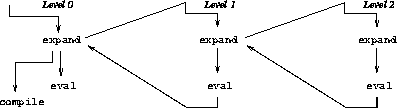# A short description of abbrevations (ako macros)

This is a short description of the macro system fully detailed in chapter 9 of my book . Alternatively, you may read the associated paper REFLECTION96.

The macro system is based on a tower of evaluator/expander as shown in the following figure:To be evaluated an expression has to be expanded before being evaluated or compiled. To define a macro ie to associate to a name a function known as the expander requires an evaluator to turn the description of a macro into an invokable function. This evaluation and the expansion itself are performed at the level above. In turn, the evaluation of the level above requires another additional level if it ever uses macros ie if macros use macros to be defined. This is the basic model. These kind of macros are called abbreviations to distinguish them from other models. Four predefined abbreviations exist:

```   (eval-in-abbreviation expression)
```

This abbreviation evaluates the ` expression ` at the level above and consider the result of it as the resulting expansion.

```   (define-abbreviation (name variables ...) body ...)
```

This abbreviation defines a new abbreviation for the current level. Whenever a use of that abbreviation is seen at the current level, the associated expander function is invoked at the level above with the associated parameters. The expander is obtained via the evaluation of ` (lambda (variables ..) body ...)` at the level above.

```   (let-abbreviation ((name variables ...) body ...) ...) expression)
```

This abbreviation defines abbreviations that are local to ``` expression```.

```   (with-aliases ((variable word) ..) expression ...)
```

This abbreviation binds locally at the level above the variables named ` variable`s to the meaning of the corresponding ``` word```s in the current level. These local bindings are only visible from the computations at the level above that appears in ` expression ..`. It is then possible to capture the meaning of variables, special forms such as ` if`, or other words such as ` else`.

## Example

Here is a small example of abbreviation: the hygienic loop-exit abbreviation:
```(with-aliases
((%call/cc call/cc)
(%lambda  lambda)
(%let     let) )
(define-abbreviation (loop . body)
(let ((loop (gensym)))
`(,%call/cc (,%lambda (exit)
(,%let ,loop () ,@body (,loop)) )) ) ) )
```
The `loop` variable is introduced hygienically while the `exit` is non-hygienically introduced so ` body` can use it to exit the loop.

Updated by Christian.Queinnec@lip6.fr
\$Id: chap9.html,v 1.11 2003/01/22 20:01:21 queinnec Exp \$